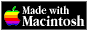THE HYPERCARD CENTER

 HyperTalk Reference
 Home » HyperTalk Reference » Operators and constants Select a destination << Home Stack < HyperTalk Reference Arithmetic operatorsComparison operatorsLogical operatorsString operatorsType and existence operatorsConstants

# Constants

### down

Equal to the literal` "down"`.

`if the mouse is down then exit repeat`

### empty

Equal to the literal` "" `(the null string)

`if it is empty then exit askTheUser`

### false

The opposite of` true`; equal to the literal` "false" `

`if the hilite of button 1 is false then mark this card`

### formFeed

Equal to` numToChar(12)`, which starts a new page in some file formats.

`write formFeed to file "My Info"`

### lineFeed

Equal to` numToChar(10)`, which starts a new line in some file formats.

`write lineFeed to file "My Info"`

### pi

Equal to the mathematical value pi to 20 decimal places:

`3.14159265358979323846`

### quote

Equal to the double quotation mark character:`"`

`get "stack" && quote & "Home" & quote --it now contains the string: stack "Home" go it`

### return

Equal to` numToChar(13)`.

```put return after field 1 write return & return to file "Data" ```

### space

Equal to` numToChar(32)` or the literal` " "`.

```if theCharacter is space then exit checkActiveText ```

### tab

Equal to` numToChar(9)`.

`write tab to file "My Info"`

### true

The opposite of` false`; equal to the literal` "true"`.

```if the hilite of button 1 is true then unmark this card ```

### up

Equal to the literal` "up"`.

`if the mouse is up then exit repeat`

### zero..ten

Equal to the numbers 0 through 10.

`go card one`

### comma

Equal to the character `","` (`numToChar = 44`)

`read from file "Data" until comma`

### colon

Equal to the character `":"` (`numToChar = 58`)

`set the itemDelimiter to colon`

Version 0.7b1 (March 24, 2022)Switch to Modern View